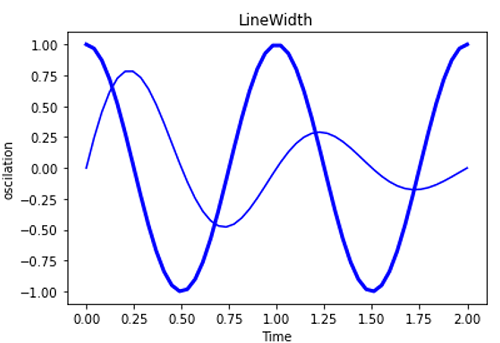# Python | Controlling the Line Width

Python | Controlling the Line Width: In this article, we are going to learn about controlling the line width and its Python implementation.
Submitted by Anuj Singh, on July 13, 2020

Line width is another property that can play a big role in basic data visualization. Bold lines have a major impact on readability than thin lines. Plotting includes sum or average line are plotting with greater line width. Because of such type of usage, matplotlib provides a feature to change linewidth.

Following are some examples of categorical plotting:

Syntax:

```matplotlib.pyplot.plot(x,y,linewidth=2.0)

# linewidth=2.0
# Width of the line
```## Python code for controlling the line width

```# Data Visualization using Python
# Controling line width

import numpy as np
import matplotlib.pyplot as plt

x1 = np.linspace(0.0, 2.0)
x2 = np.linspace(0.0, 2.0)

y1 = np.sin(2 * np.pi * x1) * np.exp(-x1)
y2 = np.cos(2 * np.pi * x2)

plt.plot(x1, y1, 'b')
plt.plot(x2, y2, 'b', linewidth=3.0)
plt.xlabel('Time')
plt.ylabel('oscilation')
plt.title('LineWidth')
plt.figure()
```

Output:

```Output is as figure
```

Languages: » C » C++ » C++ STL » Java » Data Structure » C#.Net » Android » Kotlin » SQL
Web Technologies: » PHP » Python » JavaScript » CSS » Ajax » Node.js » Web programming/HTML
Solved programs: » C » C++ » DS » Java » C#
Aptitude que. & ans.: » C » C++ » Java » DBMS
Interview que. & ans.: » C » Embedded C » Java » SEO » HR
CS Subjects: » CS Basics » O.S. » Networks » DBMS » Embedded Systems » Cloud Computing
» Machine learning » CS Organizations » Linux » DOS
More: » Articles » Puzzles » News/Updates